# Colloquium.pptx

22 de Oct de 2022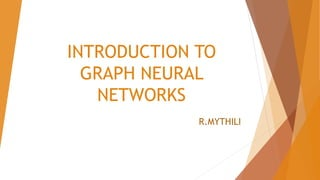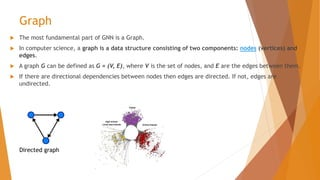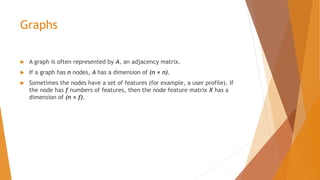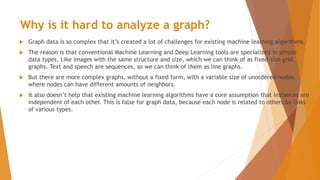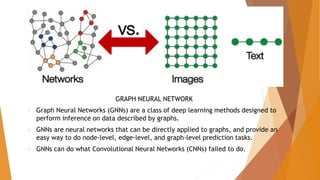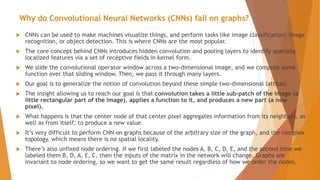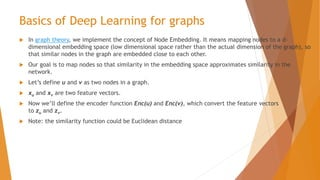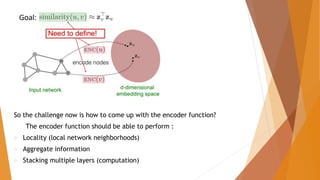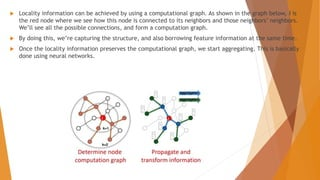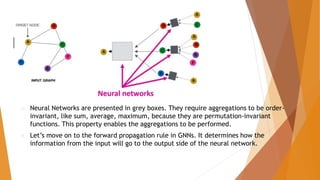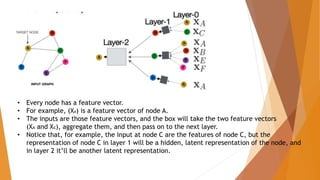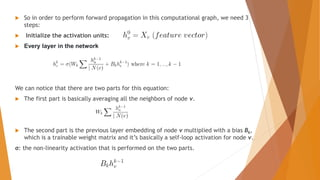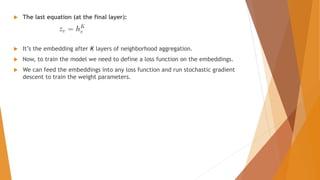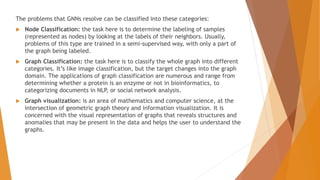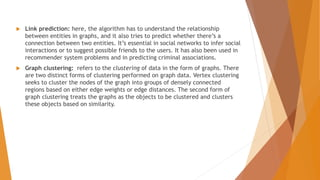1 de 16

### Colloquium.pptx

• 2. Graph  The most fundamental part of GNN is a Graph.  In computer science, a graph is a data structure consisting of two components: nodes (vertices) and edges.  A graph G can be defined as G = (V, E), where V is the set of nodes, and E are the edges between them.  If there are directional dependencies between nodes then edges are directed. If not, edges are undirected. Directed graph
• 3. Graphs  A graph is often represented by A, an adjacency matrix.  If a graph has n nodes, A has a dimension of (n × n).  Sometimes the nodes have a set of features (for example, a user profile). If the node has f numbers of features, then the node feature matrix X has a dimension of (n × f).
• 4. Why is it hard to analyze a graph?  Graph data is so complex that it’s created a lot of challenges for existing machine learning algorithms.  The reason is that conventional Machine Learning and Deep Learning tools are specialized in simple data types. Like images with the same structure and size, which we can think of as fixed-size grid graphs. Text and speech are sequences, so we can think of them as line graphs.  But there are more complex graphs, without a fixed form, with a variable size of unordered nodes, where nodes can have different amounts of neighbors.  It also doesn’t help that existing machine learning algorithms have a core assumption that instances are independent of each other. This is false for graph data, because each node is related to others by links of various types.
• 5. GRAPH NEURAL NETWORK  Graph Neural Networks (GNNs) are a class of deep learning methods designed to perform inference on data described by graphs.  GNNs are neural networks that can be directly applied to graphs, and provide an easy way to do node-level, edge-level, and graph-level prediction tasks.  GNNs can do what Convolutional Neural Networks (CNNs) failed to do.
• 6. Why do Convolutional Neural Networks (CNNs) fail on graphs?  CNNs can be used to make machines visualize things, and perform tasks like image classification, image recognition, or object detection. This is where CNNs are the most popular.  The core concept behind CNNs introduces hidden convolution and pooling layers to identify spatially localized features via a set of receptive fields in kernel form.  We slide the convolutional operator window across a two-dimensional image, and we compute some function over that sliding window. Then, we pass it through many layers.  Our goal is to generalize the notion of convolution beyond these simple two-dimensional lattices.  The insight allowing us to reach our goal is that convolution takes a little sub-patch of the image (a little rectangular part of the image), applies a function to it, and produces a new part (a new pixel).  What happens is that the center node of that center pixel aggregates information from its neighbors, as well as from itself, to produce a new value.  It’s very difficult to perform CNN on graphs because of the arbitrary size of the graph, and the complex topology, which means there is no spatial locality.  There’s also unfixed node ordering. If we first labeled the nodes A, B, C, D, E, and the second time we labeled them B, D, A, E, C, then the inputs of the matrix in the network will change. Graphs are invariant to node ordering, so we want to get the same result regardless of how we order the nodes.
• 7. Basics of Deep Learning for graphs  In graph theory, we implement the concept of Node Embedding. It means mapping nodes to a d- dimensional embedding space (low dimensional space rather than the actual dimension of the graph), so that similar nodes in the graph are embedded close to each other.  Our goal is to map nodes so that similarity in the embedding space approximates similarity in the network.  Let’s define u and v as two nodes in a graph.  xu and xv are two feature vectors.  Now we’ll define the encoder function Enc(u) and Enc(v), which convert the feature vectors to zu and zv.  Note: the similarity function could be Euclidean distance
• 8. So the challenge now is how to come up with the encoder function? The encoder function should be able to perform :  Locality (local network neighborhoods)  Aggregate information  Stacking multiple layers (computation)
• 9.  Locality information can be achieved by using a computational graph. As shown in the graph below, i is the red node where we see how this node is connected to its neighbors and those neighbors’ neighbors. We’ll see all the possible connections, and form a computation graph.  By doing this, we’re capturing the structure, and also borrowing feature information at the same time.  Once the locality information preserves the computational graph, we start aggregating. This is basically done using neural networks.
• 10.  Neural Networks are presented in grey boxes. They require aggregations to be order- invariant, like sum, average, maximum, because they are permutation-invariant functions. This property enables the aggregations to be performed.  Let’s move on to the forward propagation rule in GNNs. It determines how the information from the input will go to the output side of the neural network.
• 11. • Every node has a feature vector. • For example, (XA) is a feature vector of node A. • The inputs are those feature vectors, and the box will take the two feature vectors (XA and Xc), aggregate them, and then pass on to the next layer. • Notice that, for example, the input at node C are the features of node C, but the representation of node C in layer 1 will be a hidden, latent representation of the node, and in layer 2 it’ll be another latent representation.
• 12.  So in order to perform forward propagation in this computational graph, we need 3 steps:  Initialize the activation units:  Every layer in the network We can notice that there are two parts for this equation:  The first part is basically averaging all the neighbors of node v.  The second part is the previous layer embedding of node v multiplied with a bias Bk, which is a trainable weight matrix and it’s basically a self-loop activation for node v. σ: the non-linearity activation that is performed on the two parts.
• 13.  The last equation (at the final layer):  It’s the embedding after K layers of neighborhood aggregation.  Now, to train the model we need to define a loss function on the embeddings.  We can feed the embeddings into any loss function and run stochastic gradient descent to train the weight parameters.
• 14. The problems that GNNs resolve can be classified into these categories:  Node Classification: the task here is to determine the labeling of samples (represented as nodes) by looking at the labels of their neighbors. Usually, problems of this type are trained in a semi-supervised way, with only a part of the graph being labeled.  Graph Classification: the task here is to classify the whole graph into different categories. It’s like image classification, but the target changes into the graph domain. The applications of graph classification are numerous and range from determining whether a protein is an enzyme or not in bioinformatics, to categorizing documents in NLP, or social network analysis.  Graph visualization: is an area of mathematics and computer science, at the intersection of geometric graph theory and information visualization. It is concerned with the visual representation of graphs that reveals structures and anomalies that may be present in the data and helps the user to understand the graphs.
• 15.  Link prediction: here, the algorithm has to understand the relationship between entities in graphs, and it also tries to predict whether there’s a connection between two entities. It’s essential in social networks to infer social interactions or to suggest possible friends to the users. It has also been used in recommender system problems and in predicting criminal associations.  Graph clustering: refers to the clustering of data in the form of graphs. There are two distinct forms of clustering performed on graph data. Vertex clustering seeks to cluster the nodes of the graph into groups of densely connected regions based on either edge weights or edge distances. The second form of graph clustering treats the graphs as the objects to be clustered and clusters these objects based on similarity.